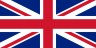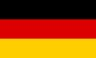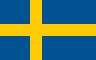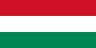GSAK (Geocaching Swiss Army Knife)Contents - Index

SqlNext (command)

SqlNext [Handle=n]

Use to iterate through a Sqlite query that has been generated with the SqlGet=Handle option of Sqlite(). For more information and example see the SqlGet()

Handle - this is the corresponding handle number (0 to 4) used in the Sqlite() function with the option SqlGet=Handle. If the Handle= parameter is omitted then the default handle of 0 is used.

Example:

 # list the names of the first 3 caches in the grid \$Data = "" \$x = 0 \$status = sqlite("sql",\$_SqlGrid, "sqlget=0") while not(\$_sqleol0)   \$x = \$x + 1   \$data = \$data + sqlget("name",0) + \$_CrLf   if \$x = 3     SqlGetClose Handle=0     Break   EndIf   sqlnext Handle=0 Endwhile cancel msg=\$data

As the default handle is 0, the Handle for SqlGetClose,SqlNext,SqlGet and \$_SqlEol is not really required. So for brevity, the previous example can also be coded as:

 # list the names of the first 3 caches in the grid \$Data = "" \$x = 0 \$status = sqlite("sql",\$_SqlGrid, "sqlget=0") while not(\$_sqleol)   \$x = \$x + 1   \$data = \$data + sqlget("name") + \$_CrLf   if \$x = 3     SqlGetClose     Break   EndIf   sqlnext Endwhile cancel msg=\$data

Alpha List         Category List